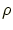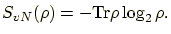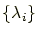Display the von Neumann entropy

Generally, the von Neumann entropy of a density matrixis defined by(14)

We use the binary logarithm (base 2) in this software. It is also written by using the eigenvaluesofas(15)

This is because a density matrixcan be diagonalized by a proper unitary transformation. Such a transformation preserves eigenvalues of. A density matrixis an Hermitian matrix and its eigenvalues are non-negative real numbers.

The command

SvN;
shows the von Neumann entropy of the current density matrix.

The command

SvNRDO(bit labels);
shows the von Neumann entropy of the reduced density operator of the qubits specified by bit labels.

root
2004-11-07